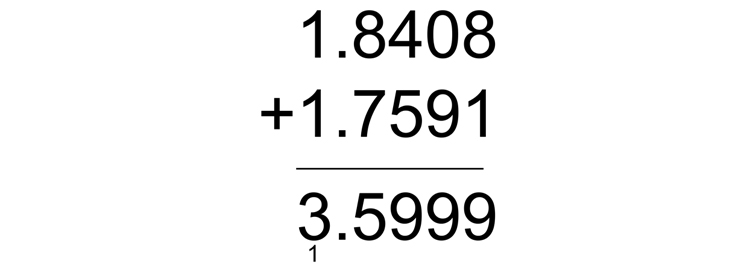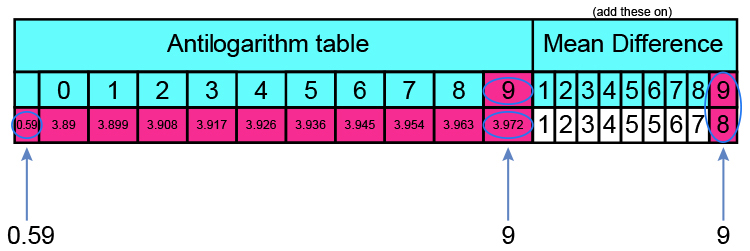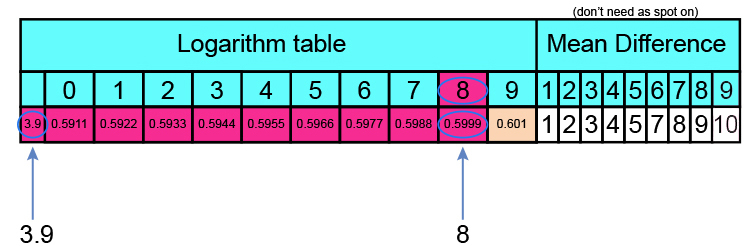# The antilog tables

69.31\times 57.43

Is the same as 10^1.8408\times 10^1.7591 (see previous pages)This equals 10^3.5999

So how do we convert 10^3.5999 back to a normal number?

We can either use

1.  Antilog tables

or

2.  log tables in reverse

## Antilog tables

Antilog 10^3.5999

Antilogs only work between 0 and 1

10^3.5999 = 10^3\times10^0.5999

10^3 = 1000

And we must look up 0.5999

Here is part of the antilog tables:3.972 + 8  (to the last digit) = 3.980

Antilog table of 0.5999 = 3.980

So 10^3.5999 = 10^3\times 10^0.5999

Or 10^3.5999 = 1000\times 3.980

 = 3980

NOTE:

There is one other way to look up the antilog figure if you don’t have an antilog table and that is to use the log tables in reverse.

Antilog tables = Log tables in reverse

## Antilog of 0.5999 but using log tables in reverse

Here is part of the log tables:0.5999 = 3.980

(So this provides the same answer)